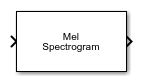# Mel Spectrogram

Extract mel spectrogram from audio

Since R2022a

•Libraries:
Audio Toolbox / Features

## Description

The Mel Spectrogram block extracts the mel spectrogram from the audio input signal. A mel spectrogram contains an estimate of the short-term, time-localized frequency content of the input signal in the mel frequency scale.

## Ports

### Input

expand all

Audio input signal, specified as a column vector or a matrix. When you specify a matrix, the block treats columns as independent audio channels.

Data Types: `single` | `double`

### Output

expand all

Mel spectrogram, returned as a matrix or 3-D array. The dimensions of spec are L-by-M-by-N, where:

• L is the number of spectra, which is determined by the Number of spectra parameter.

• M is the number of bands, which is determined by the Number of bands parameter.

• N is the number of channels in the input audio signal.

Trailing singleton dimensions are removed from the output.

This port is unnamed until you select the Output center frequencies parameter.

Data Types: `single` | `double`

Center frequencies of the bandpass filters in Hz, returned as a row vector with number of elements equal to the number of bands.

#### Dependencies

To enable this port, select the Output frequency vector parameter.

Data Types: `single` | `double`

## Parameters

expand all

Filter Bank Parameters

Number of bandpass filters, specified as a positive integer.

When you select this parameter, the block sets the Frequency range to `[0,fs/2]`, where `fs` is the sample rate. The sample rate is determined by the Inherit sample rate from input and Input sample rate (Hz) parameters.

Frequency range in Hz over which to design the auditory filter bank, specified as a two-element row vector.

#### Dependencies

To enable this parameter, clear the Auto-determine frequency range parameter.

Domain in which the block designs the filter bank, specified as `linear` or `warped`. Set the filter bank design domain to `linear` to design the bandpass filters in the linear (Hz) domain. Set the filter bank design domain to `warped` to design the bandpass filters in the warped (mel) domain.

Normalization technique used for the filter bank weights, specified as `bandwidth`, `area`, or `none`.

• `bandwidth` –– Normalize the weights of each bandpass filter by the corresponding bandwidth of the filter.

• `area` –– Normalize the weights of each bandpass filter by the corresponding area of the bandpass filter.

• `none` –– The block does not normalize the weights of the filters.

Open plot to visualize the filters in the frequency domain.

When you select this parameter, the block displays an additional output port, fvec. This port outputs the center frequencies of the bandpass filters.

Spectrogram Parameters

Analysis window applied in the time domain, specified as a real vector.

When you select this parameter, the block applies window normalization.

Overlap length of adjacent analysis windows, specified as an integer in the range [0, `windowLength`), where `windowLength` is the length of the analysis window, which is specified by Window.

When you select this parameter, the block automatically sets the FFT length to the window length, `numel(Window)`.

Number of points used to calculate the DFT, specified as a positive integer.

#### Dependencies

To enable this parameter, clear the Auto-determine FFT length parameter.

Type of spectrum, specified as `magnitude` or `power`.

Number of spectra in the spectrogram, specified as a positive integer.

Number of spectra overlapped across consecutive spectrograms, specified as an integer in the range [0, Number of spectra)

Simulation Parameters

When you select this parameter, the block inherits its sample rate from the input signal. When you clear this parameter, you specify the sample rate in the Input sample rate (Hz) parameter.

Input sample rate in Hz, specified as a real positive scalar.

#### Dependencies

To enable this parameter, clear the Inherit sample rate from input parameter.

## Block Characteristics

 Data Types `double` | `single` Direct Feedthrough `no` Multidimensional Signals `no` Variable-Size Signals `no` Zero-Crossing Detection `no`

## Version History

Introduced in R2022a

expand all